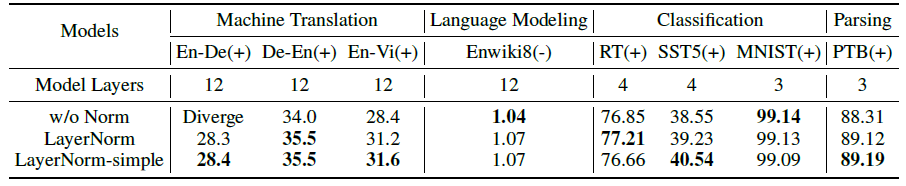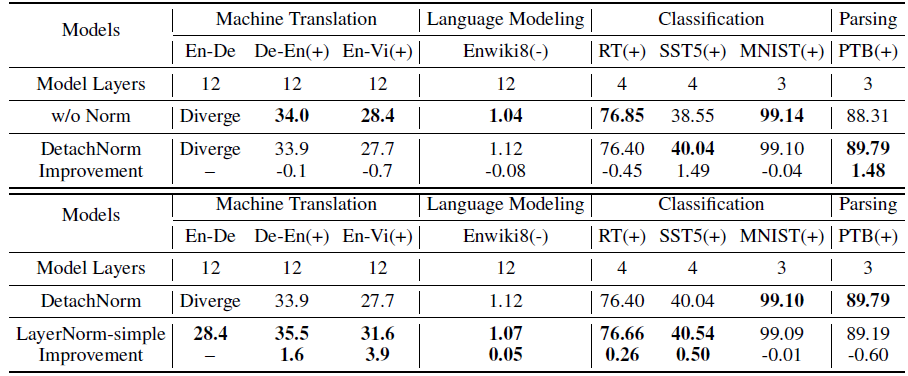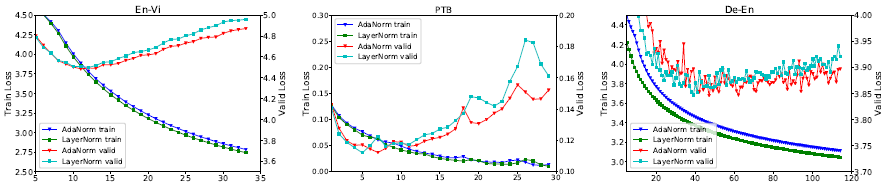## Improve LayerNorm (Layer Normalization)

Understanding and Improving Layer Normalization
AdaNorm, by Peking University
2019 NeurIPS, Over 50 Citations (Sik-Ho Tsang @ Medium)
Machine Translation, Language Model, Image Classification, Layer Normalization

• By understanding LayerNorm (Layer Normalization), a step further is made to improve LayerNorm as AdaNorm (Adaptive Normalization).

# Outline

1. LayerNorm
2. LayerNorm-simple
3. DetachNorm

# 1. LayerNorm

• Let x=(x1, x2, …, xH) be the vector representation of an input of size H to normalization layers. LayerNorm re-centers and re-scales input x as:
• where h is the output of a LayerNorm layer. ⊙ is a dot production operation. μ and σ are the mean and standard deviation of input. Bias b and gain g are parameters with the same dimension H.
• LayerNorm is a default setting in Transformer and Transformer-XL.

# 2. LayerNorm-simpleLayerNorm-simple outperforms LayerNorm on 6 datasets
• For machine translation, Transformer is re-implemented.
• For language model, 12-layer Transformer-XL is used.
• For text classification, Transformer with a 4-layer encoder is used.
• For image classification, 3-layer CNN is used.
• For parsing, MLP-based parser is used.

The bias and gain do NOT work on six out of eight datasets.

# 3. DetachNorm

• Detaching derivatives means treating the mean and variance as changeable constants, rather than variables, which do not require gradients in backward propagation.
• The function θ(.) can be seen as a special copy function, which copies the values of μ and σ into constants ^μ and ^σ.

In all, DetachNorm keeps the same forward normalization fact as LayerNorm does, but cuts offs the derivatives of the mean and variance.The derivatives of the mean and variance matter
• DetachNorm performs worse than “w/o Norm”, showing that forward normalization has little to do with the success of LayerNorm.

DetachNorm performs worse than LayerNorm-simple on six datasets. The derivatives of the mean and variance bring higher improvements than forward normalization does.

• In AdaNorm, Φ(y), a function with respect to input x, is used to replace the bias and gain with the following equation:

Unlike the bias and gain being fixed in LayerNorm, Φ(y) can adaptively adjust scaling weights based on inputs.

• To keep the training stability, some constraints are made. (1) First, Φ(y) must be differentiable. (2) Second, the average scaling weight is expected to be fixed, namely the average of Φ(y) is a constant C where C > 0. (3) Third, it is expected that the average of z is bounded, which can avoid the problem of exploding loss.
• By considering above constraints and based on Chebyshev’s Inequality, finally, Φ(y) is:
• (Please feel free to read the paper if interested for this proof.)
• Given an input vector x, the complete calculation process of AdaNorm is:
• where C is a hyper-parameter, k=1/10.
• In implementation, the gradient of C(1-ky) is detached and it is only treated as a changeable constant.
• AdaNorm outperforms LayerNorm on seven datasets, with 0.2 BLEU on En-De, 0.1 BLEU on De-En, 0.2 BLEU on En-Vi, 0.29 ACC on RT, 1.31 ACC on SST, 0.22 ACC on MNIST, and 0.11 UAC on PTB.

Unlike LayerNorm-simple only performing well on bigger models, AdaNorm achieves more balanced results.Loss curves of LayerNorm and AdaNorm on En-Vi, PTB, and De-En.
• The above figure shows the loss curves of LayerNorm and AdaNorm on the validation set of En-Vi, PTB, and De-En.
• Compared to AdaNorm, LayerNorm has lower training loss but higher validation loss. Lower validation loss proves that AdaNorm has better convergence.